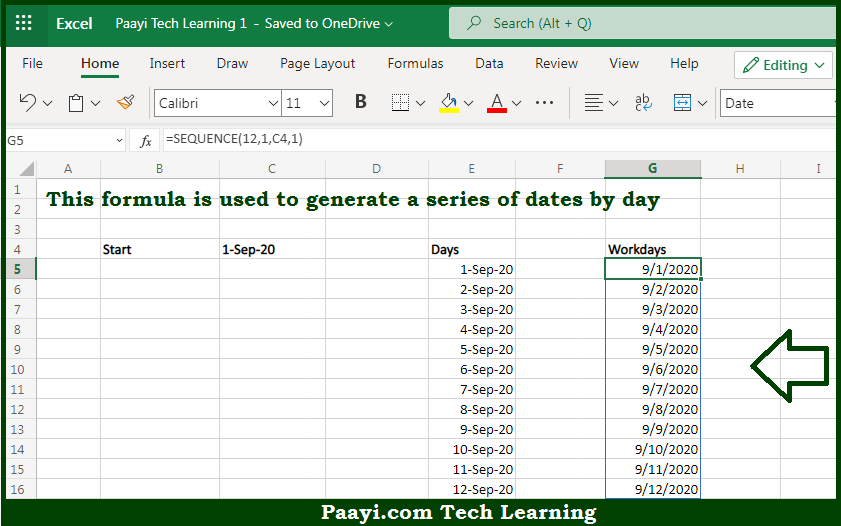# Learn How to Get Sequence of Days in Microsoft Excel

Written by | 0 Comments | 485 Views

In this article, you will learn how to evaluate things in Dynamic Arrays with formulas in Microsoft Excel using a single/combination(s) of functions. You will also know How to Get Sequence of Days and see the generic formula.

Learn How to Get Sequence of Days in Microsoft Excel

The main purpose of this formula is to generate a series of dates by day. Here we will learn how to get the sequence of days in the given data range in the workbook in Microsoft Excel. That implies, with the help of a formula based on the SEQUENCE function you can able to generate a series of dates by day. So, with the help of this formula, you can able to get the sequence of days in the given data range in the workbook in Microsoft Excel.

General Formula to Get Sequence of Days

=SEQUENCE(days,1,start_date,step)

The Explanation to Get Sequence of DaysSo we know that with the help of the given formula above you can able to generate a series of dates by day. Here we will learn how to get the sequence of days in the given data range in the workbook in Microsoft Excel. As we know that the SEQUENCE function is a dynamic array function that is used to generate multiple results. while using it by itself on the worksheet, SEQUENCE functions return an array of results that "spill" onto the worksheet in a "spill range". SEQUENCE function can also generate results in rows, columns, or rows and columns. So, with the help of this formula, you can able to generate a series of dates by day. So now you have learned how to get the sequence of days in the given data range in the workbook in Microsoft Excel.